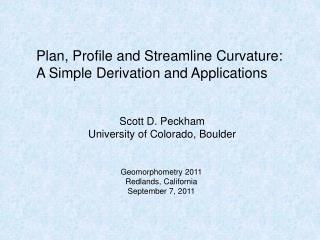Download PresentationPlan, Profile and Streamline Curvature: A Simple Derivation and Applications

# Plan, Profile and Streamline Curvature: A Simple Derivation and Applications

Download Presentation## Plan, Profile and Streamline Curvature: A Simple Derivation and Applications

- - - - - - - - - - - - - - - - - - - - - - - - - - - E N D - - - - - - - - - - - - - - - - - - - - - - - - - - -
##### Presentation Transcript

1. Plan, Profile and Streamline Curvature: A Simple Derivation and Applications Scott D. Peckham University of Colorado, Boulder Geomorphometry 2011 Redlands, California September 7, 2011

2. Fix the figure on Slope and Aspect.

3. Slope and Aspect

4. Directional Derivative Gives the rate at which a scalar field, given by F(x,y), is changing in the direction of a (local) unit vector, n hat, such as: The scalar field, F(x,y) can be any function of x and y, such as the slope, S(x,y), or aspect, phi(x,y).

5. Example 1: Profile Curvature If we compute the directional derivative with F(x,y) = S(x,y) and the norm-alized gradient of f(x,y) as n hat, we get the equation for profile curvature. That is, we get the rate at which surface slope, S(x,y) , changes as we move in the direction of grad(f), (i.e. following a streamline). Recall: Expressed in Cartesian coordinates we have: The sign of this scalar field determines whether the longitudinal profile is locally concave up, flat, or concave down at any given point (x,y).

6. Example 2: Streamline Curvature If we compute the directional derivative with F(x,y) = psi(x,y) and the normalized gradient of f(x,y) as n hat, we get the equation for streamline curvature. That is, we get the rate at which the flow direction (aspect) changes as we move in the direction of grad(f), (i.e. following a streamline). It is the inverse of a channel’s local radius of curvature, which measures how tightly a channel bends. Recall: Expressed in Cartesian coordinates we have:

7. Example 3: Plan Curvature If we compute the directional derivative with F(x,y) = phi(x,y) and the normalized perpendicular gradient of f(x,y) as n hat, we get the equation for plan curvature. This is the rate at which flow direction (aspect angle) changes as we move in the direction of grad_perp(f), (i.e. following a contour line). It is negative for channels and positive for ridges. Recall: Expressed in Cartesian coordinates we have: Note: grad_perp(f) is perpendicular and to the right of grad(f) and to the left of -grad(f). Tangential curvature is a closely-related type of normal curvature.

8. Parabolic Valley and Ridge

9. Geometric Optics Equation – Spiral Bowl All solutions to the Equation of Geometric Optics have profile and streamline curvatures equal to zero everywhere. Equation of Geometric Optics

10. Geometric Optics Equation – Meander Another solution to the Equation of Geometric Optics, so profile and streamline curvatures are equal to zero everywhere. Contour lines are “sine-generated” meander curves.

11. A “Sine Valley” Surface z(x,y) = (x/10) - Sin[y – Sin(x)]

12. Curvatures for a “Sine Valley” Surface elevation contour plot abs(streamline curvature) profile curvature streamline curvature plan curvature

13. Streamline “Rose” Surface z(r, theta) = 0.2 [ r^2/10 + r * Sin(8 theta – Sin(r)) ]

14. Plan and Profile Curvature and Laplace’s Equation Minimal Surface Equation in Cartesian Coordinates kp + S kc (1 + S^2) = 0. S^2 << 1 => Laplace eqn.

15. An Idealized Mathematical Model for Steady-State Fluvial Landforms The case where  = -1 corresponds to a surface such that steady flow over the surface has the same unit stream power everywhere. This seems to be the case that most closely matches available data. When  < 0, long profiles are always concave up.